WelcomeFunctional CategoriesRelease NotesOverviewMembers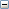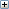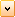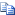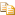IMathPoint Interface Members

The following tables list the members exposed by IMathPoint.

# Public Properties

NameDescription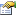ArrayDataGets or sets the array of x,y,z coordinates that describe this math point.IArrayDataGets or sets the array of x,y,z coordinates that describe this math point.
Top

# Public Methods

NameDescription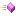AddVectorTranslates a math point by a math vector to create a new math point.ConvertToVectorConverts a math point to a math vector by using the three coordinates of the math point for the components of the math vector.IAddVectorTranslates a math point by a math vector to create a new math point.IConvertToVectorConverts a math point to a math vector by using the three coordinates of the math point for the components of the math vector.IMultiplyTransformMultiplies a math point with a math transform; the point is rotated, scaled, and then translated.IScaleScales a math point's magnitude.ISubtractGets a math vector that describes the difference between the math point magnitude from the calling math point.ISubtractVectorGets a math point that describes the difference between a math vector's magnitude from the calling math pointMultiplyTransformMultiplies a math point with a math transform; the point is rotated, scaled, and then translated.ScaleScales a math point's magnitude.SubtractGets a math vector that describes the difference between the math point magnitude from the calling math point.SubtractVectorGets a math point that describes the difference between a math vector's magnitude from the calling math point
Top

Provide feedback on this topic

* Required

 *Email: Subject: Feedback on Help Topics Page: IMathPoint Interface Members *Comment: * I acknowledge I have read and I hereby accept the privacy policy under which my Personal Data will be used by Dassault Systèmes

Print Topic

Select the scope of content to print:

x

We have detected you are using a browser version older than Internet Explorer 7. For optimized display, we suggest upgrading your browser to Internet Explorer 7 or newer.

Never show this message again
x

Web Help Content Version: API Help (English only) 2018 SP05

To disable Web help from within SOLIDWORKS and use local help instead, click Help > Use SOLIDWORKS Web Help.

To report problems encountered with the Web help interface and search, contact your local support representative. To provide feedback on individual help topics, use the “Feedback on this topic” link on the individual topic page.